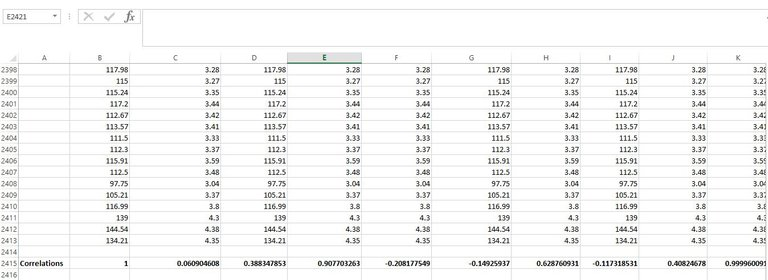# Historical Analysis of Bitcoin Correlation with Altcoins(Edited)

One of the driving force towards investment is analysis of historical records crypto assets to derive logical information. The dataset below was gotten from kaggle.com which contains information of top cryptocurrencies pulled from coinmarketcap.com.

Here is the link to dataset: Source

The data contains 28,945 entries of crypto parameters which includes the name of currency, date, open, high, low, close, volume and market capitalization of the asset as a csv file from 2013 to 2019.

## Data description

The name of currencies included as data are Bitcoin, EOS, Binance coin, Tezos, Tether, XRP, Bitcoin cash, stellar, Litecoin, Ethereum , Cardano, and Bitcoin sv.

Date represents the date information was collected, open, high, low and close represents volatility of the asset whereas volume represents the amount traded and market capitalization is the total circulating supply by the price.

However, it is of concern to find out the following questions as regards the data

## What is the correlation between Bitcoin and other altcoins?

To answer this question, the subject of correlation will be presented in this section.

Correlation between two or more assets shows the level of relationship between them. It is been measured by a coefficient which tells the rate at which increase in value of major asset (bitcoin) can influence increase in another asset (altcoins).

The coefficient of correlation could be positive 1, zero or negative 1. When it is at positive 1, the relationship is said to be strong. i.e. an increase in the price of bitcoin will influence the price of other alt coins considered individually.

When the coefficient of correlation is estimated zero indicates there is no correlation between bitcoin and alt coin individually considered.

When the coefficient of correlation is negative value then it’s said to be that an increase in price of bitcoin will lead to a decrease in the price of an altcoin.
To calculate the correlation coefficient on spreadsheet, the correlation function was used.

## Summary of bitcoin correlations

Here is a spreadsheet with the columns A to J showing the closed price of the asset for each day.Column B represents the closed price of bitcoin.

Column C represents the closed price of EOS.

Column D represents the closed price of Ethereum.

Column E represents the closed price of Litecoin.

Column F represents the closed price of Stellar.

Column G represents the closed price of Tether.

Column H represents the closed price of Tezos.

Column I represents the closed price of XRP.

Column J represents the closed price of Binance coin.

Column K represents the closed price of Cardano.

Correlation between bitcoin and itself (column B)
The correlation coefficient between bitcoin and itself in the universe is 1 and positive.
Correlation between bitcoin and EOS (column C)
The correlation between bitcoin and EOS is extremely positive (0.06). It can be concluded that volatility in bitcoin price has the weak influence on EOS volatility at the time.

Correlation between bitcoin and Ethereum (column D)
The correlation between bitcoin and Ethereum is 0.3, this is a positive correlation at weak level. Volatility in bitcoin price indirectly influenced the price of Ethereum to some extent at weak level.

Correlation between bitcoin and Litecoin (column E)
The coefficient of correlation between bitcoin and litecoin is 0.90 which is strong and positive. It can be concluded that volatility in the price of bitcoin influences the price of litecoin positively.

Correlation between bitcoin and Stellar (column F)
The coefficient of correlation between bitcoin and stellar is negative (-0.208). the relationship is said to be weak.

Correlation between bitcoin and Tether (column G)
The correlation coefficient between bitcoin and tether is weak and negative (-0.149).

Correlation between bitcoin and Tezos (column H)
The correlation between bitcoin and Tezos is strong and positive (0.62). Volatility in BTC price will influence the price of Tezos.
Correlation between bitcoin and XRP (column I)
Correlation between bitcoin and XRP is negative and weak. Volatility in bitcoin may not really affect XRP, if it does, will be in negative way.

Correlation between bitcoin and Binance coin (column J)
Correlation between bitcoin and binance coin is on a mid-level and positive (0.4). it can be concluded that volatility in bitcoin might have average influence the price of binance coin.

Correlation between bitcoin and Cardano (column K)
Correlation between bitcoin and cardano is positive and strong. Volatility in bitcoin will replicate on Cardano block chain as both are strongly correlated.

Conclusively
Bitcoin it its order of positive and strong correlation with altcoins follows the linear trend; litecoin, cardano, Tezos, binance coin. However there seem to be a linear trend of negative correlation between the following altcoins: EOS, Tether, XRP, Stellar.

Posted Using LeoFinance Beta

0
0
0.000